##### Calculus II For DummiesA Taylor polynomial approximates the value of a function, and in many cases, it’s helpful to measure the accuracy of an approximation. This information is provided by the Taylor remainder term:

f(x) = Tn(x) + Rn(x)

Notice that the addition of the remainder term Rn(x) turns the approximation into an equation. Here’s the formula for the remainder term: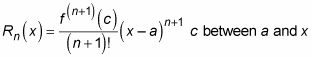It’s important to be clear that this equation is true for one specific value of c on the interval between a and x. It does not work for just any value of c on that interval.

Ideally, the remainder term gives you the precise difference between the value of a function and the approximation Tn(x). However, because the value of c is uncertain, in practice the remainder term really provides a worst-case scenario for your approximation.

The following example should help to make this idea clear, using the sixth-degree Taylor polynomial for cos x: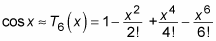Suppose that you use this polynomial to approximate cos 1: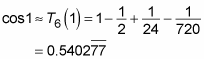How accurate is this approximation likely to be? To find out, use the remainder term:

cos 1 = T6(x) + R6(x)

Adding the associated remainder term changes this approximation into an equation. Here’s the formula for the remainder term: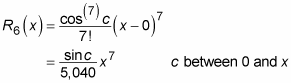So substituting 1 for x gives you: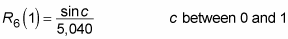At this point, you’re apparently stuck, because you don’t know the value of sin c. However, you can plug in c = 0 and c = 1 to give you a range of possible values: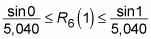Keep in mind that this inequality occurs because of the interval involved, and because that sine increases on that interval. You can get a different bound with a different interval.

This simplifies to provide a very close approximation: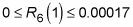Thus, the remainder term predicts that the approximate value calculated earlier will be within 0.00017 of the actual value. And, in fact,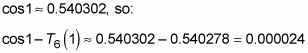As you can see, the approximation is within the error bounds predicted by the remainder term.